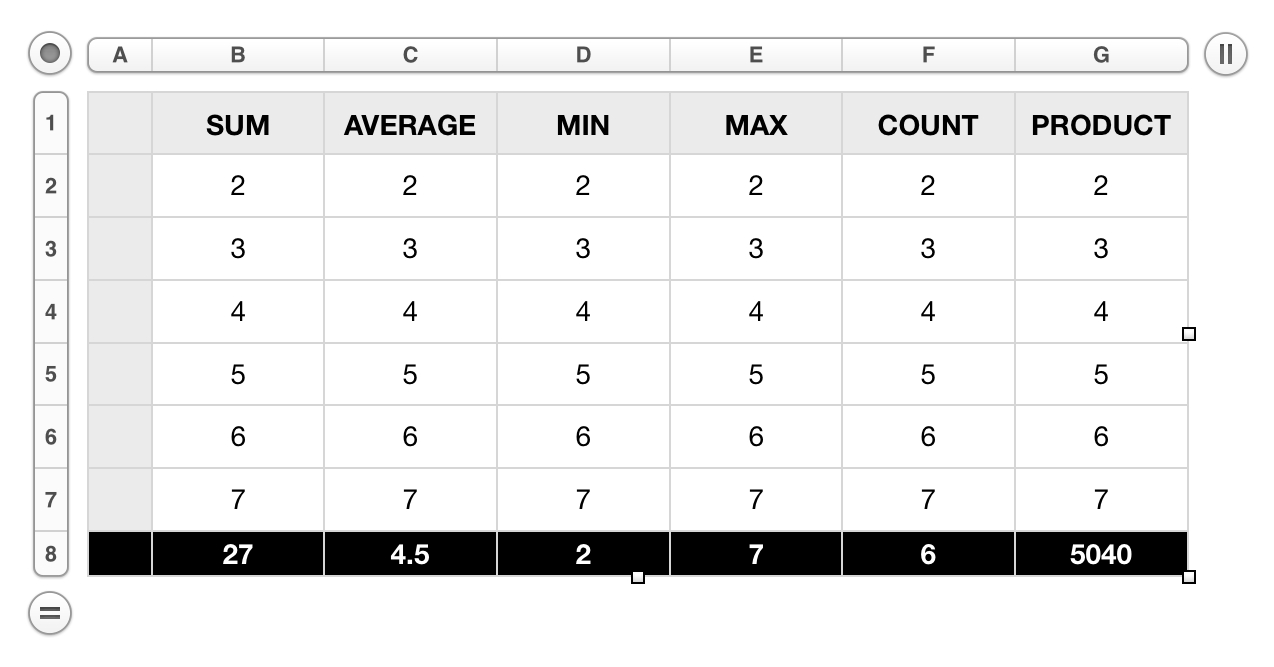Cell Formulas

The power of a spreadsheet or table derives from the ability to assign formulas to specific cells. These formulas usually involve the application of a mathematical function that uses the values of a range of cells as its input.

Constructing a Formula

In the Numbers '09 Compatibility Suite, formulas are represented as text strings beginning with an equal sign (=), followed by a function name or abbreviation, which is then followed by a range reference, comprised of the colon-delineated names of the starting and ending cells of the targeted range:

"=" & thisFunction & "(" & rangeStart & ":" & rangeEnd & ")"

For example, here are the standard function operators used in formulas:

=SUM(B2:B7)

=AVERAGE(C2:C7)

=MIN(D2:D7)

=MAX(E2:E7)

=COUNT(F2:F7)

=PRODUCT(G2:G7)

Assigning Formulas to Cells

In the Numbers '09 Compatibility Suite, there is no formula property of a cell, which could be assigned the formula as its value. Instead, a cell’s value may be set to a formula by setting it to a text string beginning with an equals sign. The text string will automatically be interpreted as a formula, whose resulting value will appear in the cell.

Function Examples Table

As a demonstration of how to assign formulas to cells, the following script example will create a new table, displaying a column whose last cell is assigned one of the standard functions using the column’s cell values as input:Here’s the script: